### Home > CC3 > Chapter Ch9 > Lesson 9.2.3 > Problem9-96

9-96.
1. For each diagram below, solve for x. Explain what method you used for each problem. Homework Help ✎

2.  a.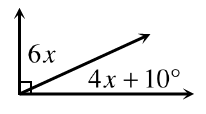b.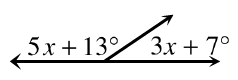c.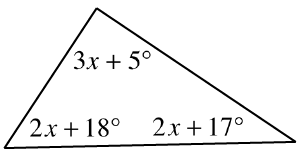d.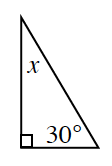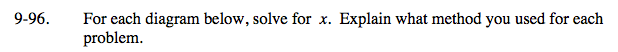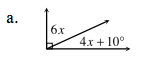The two angles are complementary, meaning they have a sum of 90°.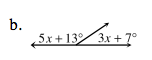The two angles are supplementary (have a sum of 180°).

x = 20°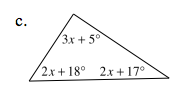According to the Angle Sum Theorem, the sum of the interior angles of a triangle is 180°.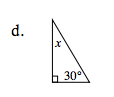How did you solve part (c)? Use the same method.

x + 30 + 90 = 180

x + 120 = 180

x = 60°